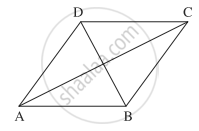# Suppose that all sides of a quadrilateral are equal in length and opposite sides are parallel. Use vector methods to show that the diagonals are perpendicular. - Mathematics and Statistics

Sum

Suppose that all sides of a quadrilateral are equal in length and opposite sides are parallel. Use vector methods to show that the diagonals are perpendicular.

#### SolutionLet ABCD be a quadrilateral in which

|bar"AB"| = |bar"BC"| = |bar"CD"| = |bar"DA"|   ....(1)

and AB || DC and AD || BC

∴ bar"AB" = bar"DC" and bar"AD" = bar"BC"    ...(2)

Now, bar"AC" = bar"AB" + bar"BC"

and bar"BD" = bar"BA" + bar"AD" = - bar"AB" + bar"BC"    ...[By(2)]

= bar"BC" - bar"AB"

∴ bar"AC".bar"BD" = (bar"AB" + bar"BC").(bar"BC" - bar"AB")

= bar"AB".(bar"BC" - bar"AB") + bar"BC" . (bar"BC" - bar"AB")

= bar"AB".bar"BC" - bar"AB".bar"AB" + bar"BC".bar"BC" - bar"BC".bar"AB"

= |bar"BC"|^2 - |bar"AB"|^2   ....[∵ bar"AB".bar"BC" = bar"BC".bar"AB"]

= 0      ...[By(1)]

∵ bar"AC", bar"BD" are non-zero vectors

∴ bar"AC" is perpendicular to bar"BD"

Hence, the diagonals are perpendicular.

Concept: Vector Product of Vectors (Cross)
Is there an error in this question or solution?